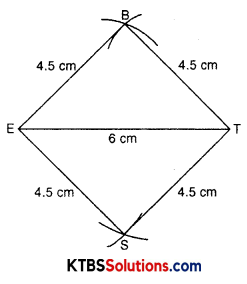# KSEEB Solutions for Class 8 Maths Chapter 4 Practical Geometry Ex 4.1

## KSEEB Solutions for Class 8 Maths Chapter 4 Practical Geometry Ex 4.1

Question 1.
AB = 4.5 cm
BC = 5.5 cm
CD = 4.4 cm
AC = 7 cm

JU = 3.5 cm
UM = 4 cm
MP = 5 cm
PJ = 4.5 cm
PU = 6.5 cm(iii) Parallelogram MORE
OR = 6 cm
RE = 4.5 cm
EO = 7.5 cm

(iv) Rhombus BEST
BE = 4.5 cm
ET = 6 cm
Solution:
(i) A rough sketch will help us to draw a quadrilateral easily: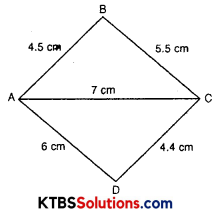Steps of construction:

1. Take a line segment AC = 7 cm.
2. Draw an arc of radius 4.5 cm taking A as centre.
3. Draw an arc of radius 5.5 cm taking C as centre.
4. These two arcs intersect, let this point be B.
5. Now, ABC is a triangle.
6. Repeat the procedure to draw ∆ADC by taking sides AD = 6 cm and CD = 4.4 cm
7. ABCD is a required quadrilateral.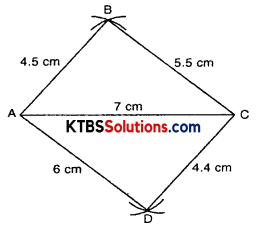(ii) Draw a rough sketch of quadrilateral JUMP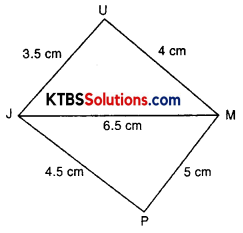Steps of construction:

1. Take a line segment JM = 6.5 cm.
2. Draw an arc of radius 3.5 cm taking J as centre.
3. Draw an arc of radius 4 cm taking M as centre.
4. These two arcs intersect each other at U.
5. Now, JUM is a triangle.
6. Repeat the procedure to draw ∆JPM by taking sides MP = 5 cm and JP = 4.5 cm
7. JUMP is a required quadrilateral.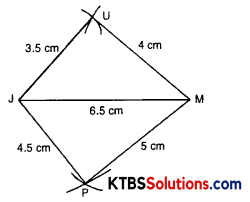(iii) Draw a rough sketch of parallelogram MORE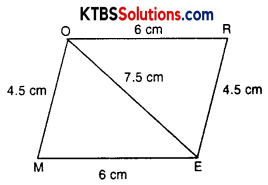In a parallelogram opposite sides are equal.
∴ OR = ME = 6 cm and RE = OM = 4.5 cmSteps of construction:

1. Draw a line segment OE = 7.5 cm.
2. Draw an arc of radius 6 cm, taking 0 as centre.
3. Draw an arc of radius 4.5 cm, taking E as centre.
4. Both these arcs intersect at R.
5. Now, ORE is a triangle.
6. Repeat the procedure by taking ME = 6 cm and OM = 4.5 cm.
7. MORE is a required parallelogram.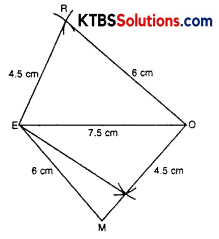(iv) Draw a rough sketch of rhombus BEST. In a rhombus all sides are equal.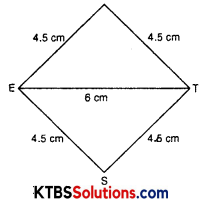Steps of construction:

1. Draw a line segment ET = 6 cm.
2. Taking radius 4.5 cm, draw an arc from E.
3. Taking radius 4.5 cm, draw an arc from T also.
4. Both these arcs intersect at B.
5. BET is a triangle.
6. Repeat the procedure to draw ∆SET by taking ES = 4.5 and ST = 4.5.
7. BEST is a required rhombus.